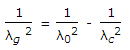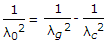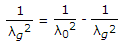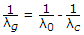# Electronics and Communication Engineering - Microwave Communication

### Exercise :: Microwave Communication - Section 8

26.

Consider the following statements about hybrid ring

1. Ports 1 and 2 are decoupled.
2. Port 3 and 4 are coupled.
3. A signal entering either port 1 or 2 splits equally between ports 3 and 4.
4. A signal entering either port 3 or 4 splits equally between ports 1 and 2.
Which of the above are correct?

 A. 1, 2, 3 and 4 B. 1, 3 and 4 C. 1 and 2 D. 2, 3 and 4

Explanation:

No answer description available for this question. Let us discuss.

27.

TM01 mode in circular waveguide is analogous to

 A. TM10 mode in square waveguide B. TM11 mode in square waveguide C. TM20 mode in square waveguide D. TM02 mode in square waveguide

Explanation:

No answer description available for this question. Let us discuss.

28.

In microwave system, waveguide twists

 A. change the plane of polarization of a propagating wave B. change the direction of a propagating wave C. change the mode of a propagating wave D. both (b) and (c)

Explanation:

No answer description available for this question. Let us discuss.

29.

Guide wavelength (λg), cutoff wavelength (λc) and free space wavelength (λ0) of a waveguide are related as

 A.B.C.D.Explanation:

No answer description available for this question. Let us discuss.

30.

A 'rat race' is a

 A. hybrid junction for microwaves B. a microwave oscillator C. a microwave amplifier D. a microwave antenna